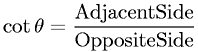Equations > Trigonometry > Trigonometric Definitions > Cotangent Definition for a Right Triangle

### Cotangent Definition for a Right TriangleLatex Code:

MathML Code:

 $\mathrm{cot}\theta =\frac\mathrm{Adjacent}\mathrm{Side}\mathrm{Opposite}\mathrm{Side}$

MathType 5.0: